Test: Progression (AP And GP)- 3

# Test: Progression (AP And GP)- 3

Test Description

## 15 Questions MCQ Test CSAT Preparation for UPSC CSE | Test: Progression (AP And GP)- 3

Test: Progression (AP And GP)- 3 for Banking Exams 2022 is part of CSAT Preparation for UPSC CSE preparation. The Test: Progression (AP And GP)- 3 questions and answers have been prepared according to the Banking Exams exam syllabus.The Test: Progression (AP And GP)- 3 MCQs are made for Banking Exams 2022 Exam. Find important definitions, questions, notes, meanings, examples, exercises, MCQs and online tests for Test: Progression (AP And GP)- 3 below.
Solutions of Test: Progression (AP And GP)- 3 questions in English are available as part of our CSAT Preparation for UPSC CSE for Banking Exams & Test: Progression (AP And GP)- 3 solutions in Hindi for CSAT Preparation for UPSC CSE course. Download more important topics, notes, lectures and mock test series for Banking Exams Exam by signing up for free. Attempt Test: Progression (AP And GP)- 3 | 15 questions in 15 minutes | Mock test for Banking Exams preparation | Free important questions MCQ to study CSAT Preparation for UPSC CSE for Banking Exams Exam | Download free PDF with solutions
 1 Crore+ students have signed up on EduRev. Have you?
Test: Progression (AP And GP)- 3 - Question 1

### A group of friends have some money which was in an increasing GP. The total money with the first and the last friend was Rs 66 and the product of the money that the second friend had and that the last but one friend had was Rs 128. If the total money with all of them together was Rs 126, then how many friends were there?

Detailed Solution for Test: Progression (AP And GP)- 3 - Question 1

The sum of money with the first and the last friend = 66. This can be used as a hint. Let us assume the first friend was having Rs 2 and the last friend was having Rs 64. So, the money can be in the sequence 2, 4, 8, 16, 32, 64. It satisfies the given conditions. Alternatively, this can be done by using the formula for tn of GP also.

Test: Progression (AP And GP)- 3 - Question 2

### The AM o f two given positive numbers is 2. I f the larger number is increased by 1, the GM of the numbers becomes equal to the AM of the given numbers. Then the HM of the given numbers is

Detailed Solution for Test: Progression (AP And GP)- 3 - Question 2

Let the numbers be a and b. let a be the larger.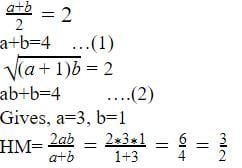Test: Progression (AP And GP)- 3 - Question 3

### A GP consists of 1000 terms. The sum of the terms occupying the odd places is Pj and the sum of the terms occupying the even places is P2. Find the common ratio of this GP.

Detailed Solution for Test: Progression (AP And GP)- 3 - Question 3

Take any small sample of 4 terms of any GP viz. 1, 2, 4 and 8. Its common ratio = 2 = (8+2)/(1 +4) And this will be true for any number of terms as long as we are taking total number of terms as even(in this question, it is given as 1000). However, if the total number of terms is given as odd, we cannot find the common ratio in this way, because the number of odd terms ≠ number of even terms.

Test: Progression (AP And GP)- 3 - Question 4

If the roots of x3 - 12x2 + 39x - 28 = 0 are in an AP then their common difference is

Detailed Solution for Test: Progression (AP And GP)- 3 - Question 4

Factorize the equation and we get ( x - 1 ) ( x - 4 ) ( x - 7 )

Test: Progression (AP And GP)- 3 - Question 5

If the nth term of AP is p and the mth term of the same AP is q, then (m + n)th term of AP is

Detailed Solution for Test: Progression (AP And GP)- 3 - Question 5

Tn = p = a + (n - l ) d

Tm = q = a + (m - l ) d

Add the two and solve through the options given.

Test: Progression (AP And GP)- 3 - Question 6

If a, b and c are positive integers, then find the product of (a + b) (b + c) (c + a).

Detailed Solution for Test: Progression (AP And GP)- 3 - Question 6

Put a= l, b = 2 c = 3 and check through options.

Test: Progression (AP And GP)- 3 - Question 7

The mid-points of the adjacent sides of a square are joined. Again the mid-points of the adjacent sides of the newly formed figure are connected and this process is repeated again and again. Calculate the sum of the areas of all such figures given that the diagonal of outermost square is 6√2cm.

Detailed Solution for Test: Progression (AP And GP)- 3 - Question 7

The side of the outermost square is 6 cm. Area of the square one in G.P with first term 36 and common ratio = 1/2

Test: Progression (AP And GP)- 3 - Question 8

There are three numbers in arithmetic progression. If the two larger numbers are both increased by one, then the resulting numbers are prime. The product of these two primes and the smallest of the original numbers is 598. Find the sum of the three numbers.

Detailed Solution for Test: Progression (AP And GP)- 3 - Question 8

Let three numbers are 2,12,22
Two larger numbers are increased by 1 : 13,23
New three numbers : 2,13,23
Product of these numbers is equal to 598.
=> 2*13*23 = 598
Sum of the three numbers = 2+13+23
= 36

Test: Progression (AP And GP)- 3 - Question 9

In how many ways can we select three natural numbers out of the first 10 natural numbers so that they are in a geometric progression with the common ratio greater than 1?

Detailed Solution for Test: Progression (AP And GP)- 3 - Question 9

1,2,4 is GP with first term 1 and common ratio 2(>1).
1,3,9 is GP with first term 1 and common ratio 3(>1).
2,4,8 is GP with first term 2 and common ratio 2(>1).
Only 3 ways.

Test: Progression (AP And GP)- 3 - Question 10

If the sum of the first 2n terms of the AP 2, 5, 8... is equal to the sum of first n terms of the AP 57, 59, 6 . . . , then what is the value of nl

Detailed Solution for Test: Progression (AP And GP)- 3 - Question 10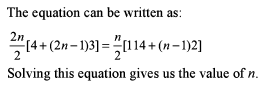Test: Progression (AP And GP)- 3 - Question 11

Given that (m +1l)th, (n + 1)th and (r +1l)th term of an AP are in GP and m , n , r are in HP, then find the ratio of the first term of the AP to its common difference in terms of n.

Detailed Solution for Test: Progression (AP And GP)- 3 - Question 11

Since the (m + 1)th, (n + 1)th and (r + 1)th term of an A.P are in G.P. so,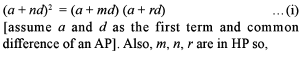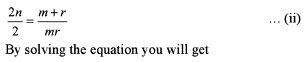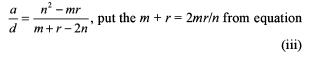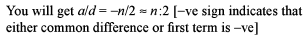Test: Progression (AP And GP)- 3 - Question 12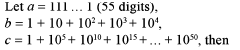Detailed Solution for Test: Progression (AP And GP)- 3 - Question 12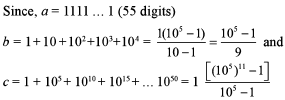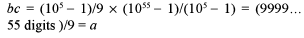Test: Progression (AP And GP)- 3 - Question 13

If a geometric mean of two non-negative numbers is equal to their harmonic mean, then which of the following is necessarily true?I. One of the numbers is zero.II. Both the numbers are equal.III. One of the numbers is one.

Detailed Solution for Test: Progression (AP And GP)- 3 - Question 13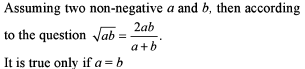Test: Progression (AP And GP)- 3 - Question 14

A series in which any term is the sum of the preceding two terms is called a Fibonacci series. The first two terms are given initially and together they determine the entire series. If the difference of the squares of the ninth and the eighth terms of a Fibonacci series is 715 then, what is the 12th term of that series?

Detailed Solution for Test: Progression (AP And GP)- 3 - Question 14

The series is like 1, 1, 2, 3, 5, 8, 13, 21, 34, 55, 89, 144...
The difference is 715 and the 12th term is 144.

Test: Progression (AP And GP)- 3 - Question 15

The first term of an AP = the common ratio of a GP and the first term of the GP = common difference of the AP. If the sum of the first two terms of the GP is equal to the sum of the first 2 terms of the AP, then the ratio of the first term of the GP to the first term of an AP is

Detailed Solution for Test: Progression (AP And GP)- 3 - Question 15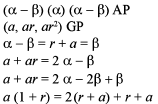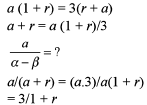This is independent of the first term of GP.

## CSAT Preparation for UPSC CSE

72 videos|64 docs|92 tests
 Use Code STAYHOME200 and get INR 200 additional OFF Use Coupon Code
Information about Test: Progression (AP And GP)- 3 Page
In this test you can find the Exam questions for Test: Progression (AP And GP)- 3 solved & explained in the simplest way possible. Besides giving Questions and answers for Test: Progression (AP And GP)- 3, EduRev gives you an ample number of Online tests for practice

## CSAT Preparation for UPSC CSE

72 videos|64 docs|92 tests